Lakhmir Singh & Manjit Kaur: Motion, Solutions- 1

# Lakhmir Singh & Manjit Kaur: Motion, Solutions- 1 - Science Class 9

Page No - 19

Question 1:
Is displacement a scalar quantity ?
Solution :
No, displacement is a vector quantity.

Question 2:
State whether distance is a scalar or a vector quantity.
Solution :
Distance is a scalar quantity.

Question 3:
Change the speed of 6 m/s into km/h.
Solution :
6 m/s
= 6 x (3600/1000) km/hr = 21.6km/hr

Question 4:
What name is given to the speed in a specified direction ?
Solution :
Speed of a body in a specified direction is called velocity.

Question 5:
Give two examples of bodies having non-uniform motion.
Solution :
(a) Motion of a bus on a road
(b) Motion of a racing horse

Question 6:
Name the physical quantity obtained by dividing ‘Distance travelled’ by ‘Time taken’ to travel that distance.
Solution :
Speed is defined as the distance travelled per unit time.

Question 7:
What do the following measure in a car ?
(a) Speedometer (b) Odometer

Solution :
(a)The speedometer of a car measures instantaneous speed of the car.
(b)Odometer is a device used to record the distance travelled by the car.

Page No:20

Question 8:
Name the physical quantity which gives us an idea of how slow or fast a body is moving.
Solution :
Speed gives an idea of how slow or fast a body is moving.

Question 9:
Under what conditions can a body travel a certain distance and yet its resultant displacement be zero ?
Solution :
When the body comes back to its starting point, it has zero resultant displacement but covers a certain non-zero distance.

Question 10:
In addition to speed, what else should we know to predict the position of a moving body ?
Solution :
In addition to speed, we should know the direction in which the body is moving.

Question 11:
When is a body said to have uniform velocity ?
Solution :
When a body covers equal distances in equal intervals of time in a particular direction however small or big the time interval may be, the object is said to have uniform velocity.

Question 12:
Under which condition is the magnitude of average velocity equal to average speed ?
Solution :
When the object moves in a single straight line, t he magnitude of average velocity equal to average speed.

Question 13:
Which of the two can be :zero under certain conditions : average speed of a moving body or average velocity of a moving body ?
Solution :
Average velocity of a moving body can be zero.

Question 14:
Give one example of a situation in which a body has a certain average speed but its average velocity is zero.
Solution :
Motion of a boy from his home to shop (in one direction) and back to home (in its reverse direction) is an example of a situation in which a body has a certain average speed but its average velocity is zero.

Question 15:
What is the acceleration of a body moving with uniform velocity ?
Solution :
When a body is moving with uniform velocity, its acceleration is zero.

Question 16:
What is the other name of negative acceleration ?
Solution :
Negative acceleration is also called retardation.

Question 17:
Name the physical quantity whose SI unit is :
(a) m/s (b) m/s
2
Solution :
(a) Speed (or Velocity)
(b) Acceleration

Question 18:
What type of motion is exhibited by a freely falling body ?
Solution :
Uniformly accelerated motion

Question 19:
What is the SI unit of retardation ?
Solution :
S.I. unit of retardation is m/s2.

Question 20:
Fill in the following blanks with suitable words :
(a) Displacement is a…………………… quantity whereas distance is a………………………….
(b) The physical quantity which gives both, the speed and direction of motion of a body is called its………………………..
(c) A motorcycle has a steady……………. of 3 m/s2. This means that every………………. its…………….. increases by………….
(d) Velocity is the rate of change of……………………… It is measured in .
(e) Acceleration is the rate of change of………………. It is measured in

Solution :
(a) vector, scalar
(b) velocity
(c) acceleration, second, velocity, 3m/s
(d) displacement, m/s
(e) velocity, m/s2

Question 21:
What type of motion, uniform or non-uniform, is exhibited by a freely falling body ? Give reason for your answer.
Solution :
A freely falling body has non-uniform motion because it covers smaller distances in the initial ‘1 second’ intervals and larger distances in the later ‘1 second’ intervals, i.e., it covers unequal distances in equal intervals of time.

Question 22:
State whether speed is a scalar or a vector quantity. Give reason for your choice.
Solution :
Speed is a scalar quantity as it has magnitude only, it has no specified direction.

Question 23:
Bus X travels a distance of 360 km in 5 hours whereas bus Y travels a distance of 476 km in 7 hours. Which bus travels faster ?
Solution :
For bus X,
Speed= Distance/Time
Speed=360/5=72km/h
For bus Y,
Speed= Distance/Time
Speed=476/7=68 km/h
Speed of bus X is more than that of bus Y. Hence, bus X travels faster.

Question 24:
Arrange the following speeds in increasing order (keeping the least speed first):

1. An athlete running with a speed of 10 m/s.
2. A bicycle moving with a speed of 200 m/min.
3. A scooter moving with a speed of 30 km/h.

Solution :
Speed of athelete = 10 m/s
Speed of bicycle = 200 m/min = 200/60 m/s = 3.33 m/s
Speed of scooter = 30 km/h = 30000/3600 m/s = 8.33 m/s
3.33 m/s < 8.33 m/s < 10 m/s
i.e. 200 m/min < 30 km/h < 10 m/s

Question 25:
(a) Write the formula for acceleration. Give the meaning of each symbol which occurs in it.
(b) A train starting from Railway Station attains a speed of 21 m/s in one minute. Find its acceleration.

Solution :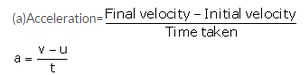(b) u = 0 m/s

v = 21m/s

Time, t =  1 min = 60 sec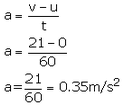Question 26:
(a) What term is used to denote the change of velocity with time ?
(b) Give one word which means the same as ‘moving with a negative acceleration’.
(c) The displacement of a moving object in a given interval of time is zero. Would the distance travelled by the object also be zero ? Give reason for your answer.

Solution :
(a) Acceleration
(b) Retardation
(c) No, because if a body takes a round trip such that its final position is same as the starting position, then the displacement of the body is zero but the distance travelled is non-zero.

Question 27:
A snail covers a distance of 100 metres in 50 hours. Calculate the average speed of snail in km/h.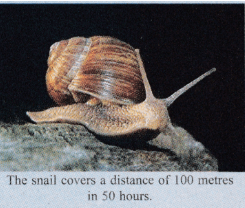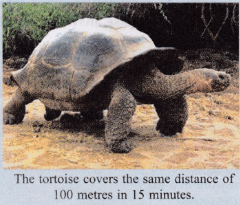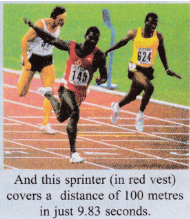Solution :
Average speed = Total distance travelled/ Total time taken
Total distance travelled = 100m = 0.1 km; Total time taken = 50 hr
Average speed= 0.1/50=0.002km/h

The document Lakhmir Singh & Manjit Kaur: Motion, Solutions- 1 | Science Class 9 is a part of the Class 9 Course Science Class 9.
All you need of Class 9 at this link: Class 9

## Science Class 9

66 videos|355 docs|97 tests

## FAQs on Lakhmir Singh & Manjit Kaur: Motion, Solutions- 1 - Science Class 9

 1. What is motion?Ans. Motion is the change in position of an object with respect to its surroundings in a given time interval. It can be described in terms of distance, displacement, speed, velocity, and acceleration.
 2. How is motion measured?Ans. Motion can be measured using various physical quantities such as distance, displacement, speed, velocity, and acceleration. Distance and displacement are measured in meters (m), speed and velocity are measured in meters per second (m/s), and acceleration is measured in meters per second squared (m/s^2).
 3. What is the difference between speed and velocity?Ans. Speed is a scalar quantity that only considers the magnitude of motion, while velocity is a vector quantity that considers the magnitude and direction of motion. In other words, speed tells us how fast an object is moving, while velocity tells us how fast an object is moving and in which direction.
 4. How can we calculate average speed?Ans. Average speed is calculated by dividing the total distance covered by an object by the total time taken. It can be represented by the formula: Average speed = Total distance / Total time.
 5. What is the difference between average speed and instantaneous speed?Ans. Average speed is calculated over a specific time interval and considers the total distance covered, while instantaneous speed refers to the speed of an object at a particular instant of time. Instantaneous speed can be found by calculating the magnitude of the instantaneous velocity at that instant.

## Science Class 9

66 videos|355 docs|97 testsExplore Courses for Class 9 examSignup to see your scores go up within 7 days! Learn & Practice with 1000+ FREE Notes, Videos & Tests.
10M+ students study on EduRev
Track your progress, build streaks, highlight & save important lessons and more!
Related Searches

,

,

,

,

,

,

,

,

,

,

,

,

,

,

,

,

,

,

,

,

,

,

,

,

;